|

# 古典欧式+新中式风格装修3室2厅2衣帽间

设计风格：古典欧式风格、新中式风格

装修材料：新西米、伯利黄、黑金花石材、木线条、皮革、银镜、做旧镜面、硬包、壁纸、铜条、木地板、地毯等

设计理念：行走在这个东西文化交融的空间中，仿佛穿越了时光的影迹，跨域了文化的藩篱。仪式厅是入门的第一道风景，处于整体空间的中心区域，功能设计的灵感来自东方古老宅邸“仪式厅”的演变。由罗马万神殿穹顶衍生出“方格叠级”的吊顶形式，在不同空间的墙、地面设计上不断延伸。空间整体采用了东方传统的“中轴对称式”布局。

PART1：客厅及会客厅设计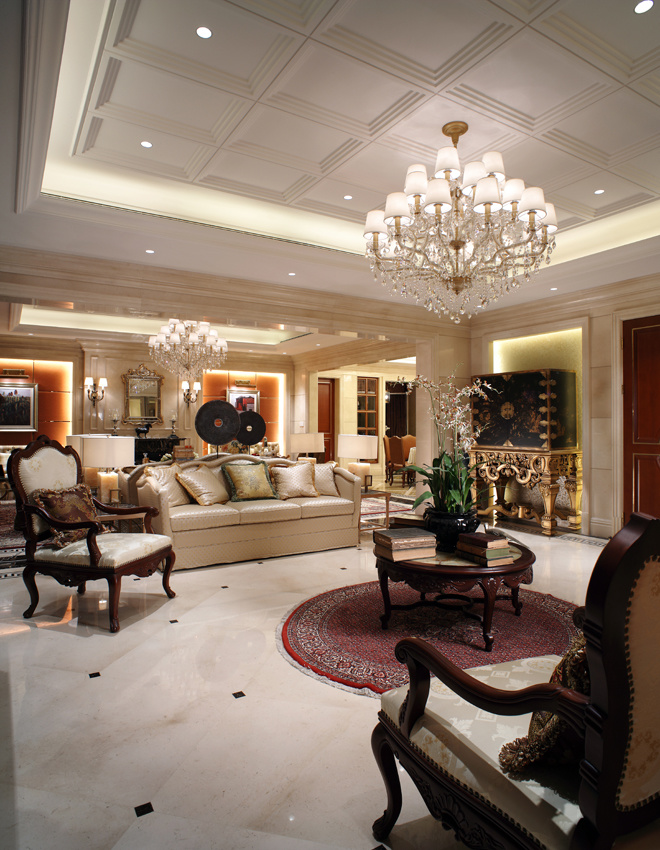设计TIPS：客厅与仪式厅设计“双厅概念”，相互贯通、融为一体，既独立又相连，多人沙发、茶几、壁炉垂直布局于一条轴线，同时也处于整个“双厅区域”的中心位置。设计TIPS：这种东方传统的“中轴对称式”布局方法，在这个整体奢华气息浓郁的欧式空间中，展现了东方文明的庄严气息。设计TIPS：奢华的水晶吊灯也是欧式风格装修中不可或缺的设计元素，颜色常见白色、黄色。设计TIPS：颜色鲜艳、花团锦绣的超大型地毯与红木茶几，一西一中两种装修风格相得益彰。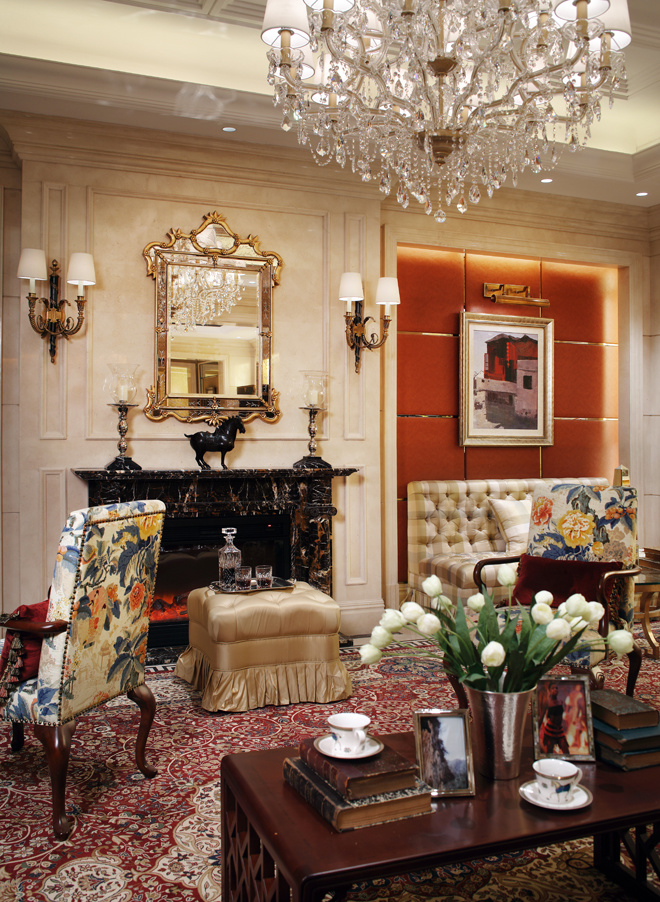设计TIPS：墙面材质采用了石材，软包，壁纸，镜子等，与家庭室外小走道形成穿透空间，与客厅壁炉两侧软包墙面形成完美对应，虚实互补。设计TIPS：整体陈设元素均展现了文化之间的交融，在彼此特种中寻求一个恰当的结合点，并融入了现代时尚的设计元素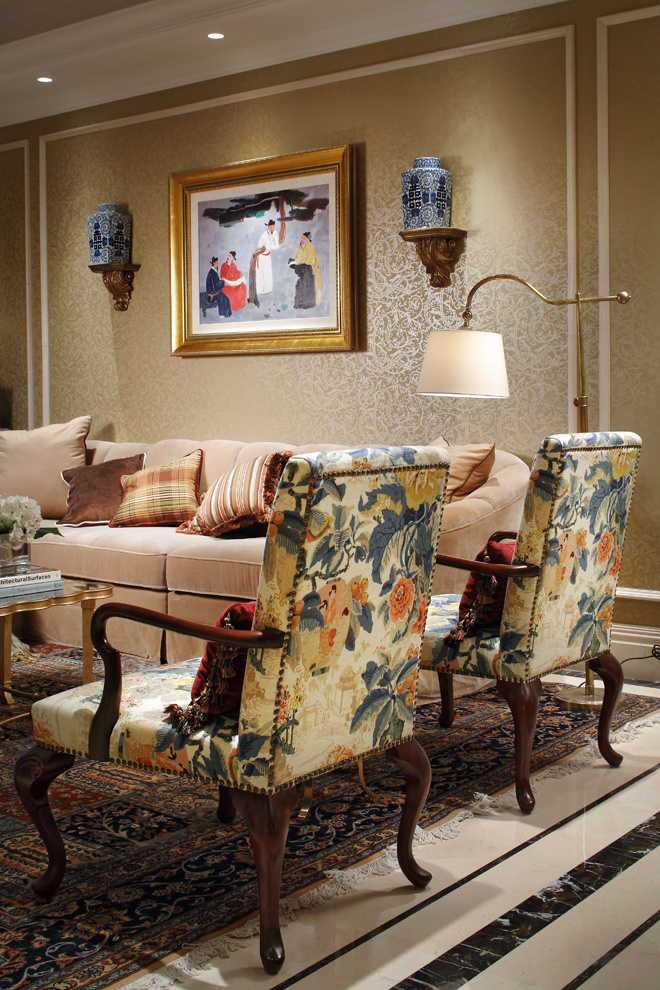设计TIPS：客厅的立面运用了西方的绘画技法表现东方文化题材的画品。

51图晒1套房子的25种不同装修风格案例

76㎡温馨和风小户型 日本夫妇教你装出古朴范儿

8款乡村风格厨房案例 田园风厨房装修效果图

19图晒300平地中海+乡村混搭风格装修样板间

105平巧设计变3室2厅2卫1衣帽间北欧豪宅

X

PART2：餐厅设计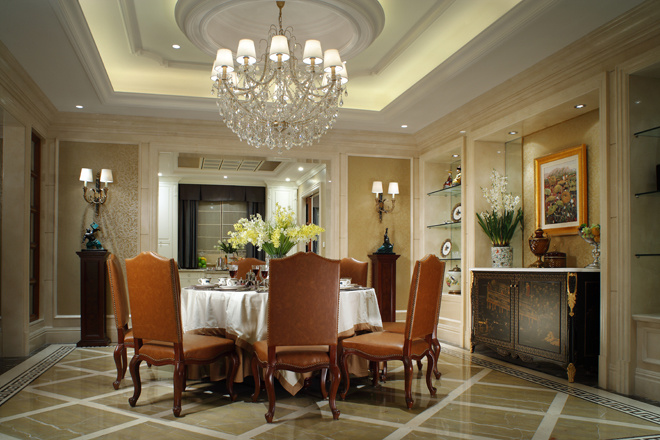设计TIPS：穿过“双厅”，右侧进入餐厅，餐厅前方是西厨。同样的“中轴对称式”布局贯穿在这个动态空间。“客厅”、“餐厅”、“西厨”处在一条中轴线上，空间流线清晰。厨房墙面与地面材质的设计手法是客厅与仪式厅的延续。餐厅右侧墙面设计了一组酒柜，与备餐柜完美结合为一个整体。深色实木的“中式备餐柜”在这个优雅、奢华的餐厅设计中透出沉稳东方气质。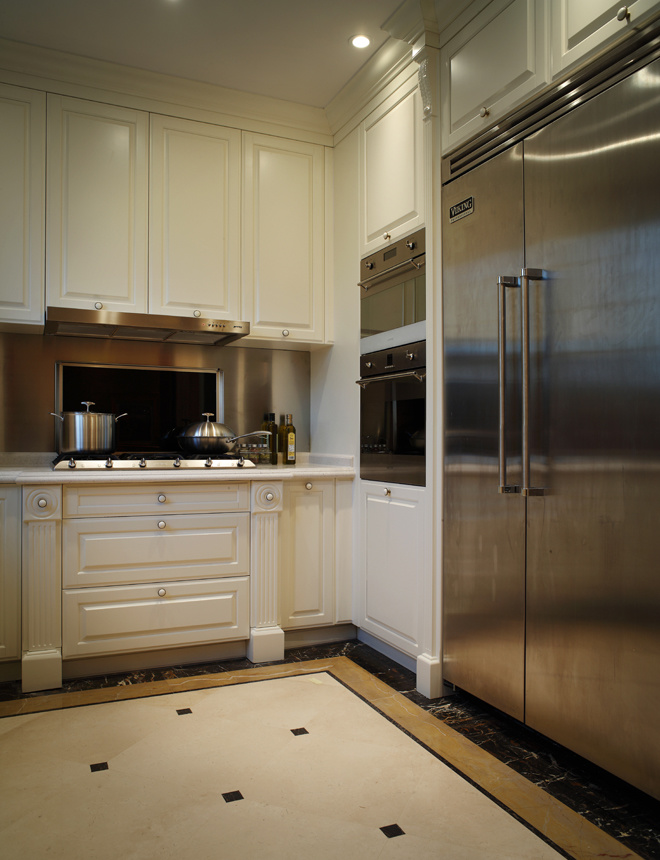设计TIPS：

白色橱柜配以银色灶具及双开门冰箱，简洁清爽的厨房让人眼前一亮。餐厅左侧是以一扇通透的玻璃隔断所设计了休息区，“玻璃”材质模糊了两个区域之间的界定，两个区域为一个整体。

PART3：休息室设计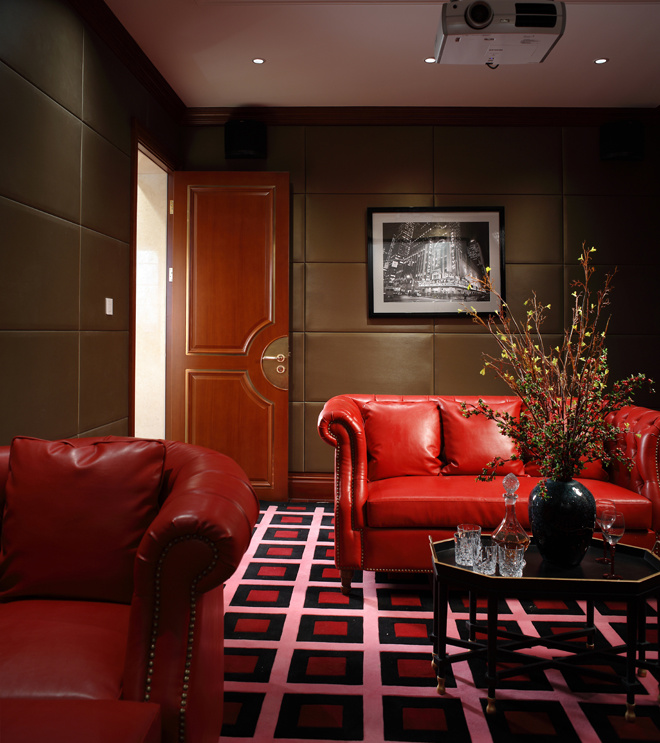设计TIPS：经过入门处的一条小走道，进入了主人卧室、儿童房、书房、休息室等相对私密的空间，与餐厅相比较，整体空间呈现出“动静结合”的状态。休息室中红色皮质沙发、经典红白黑三色地砖、皮革软包墙面、黑色多边形茶几。与三两密友浅酌共赏经典大片，多么惬意的休闲时光啊。

PART4：书房设计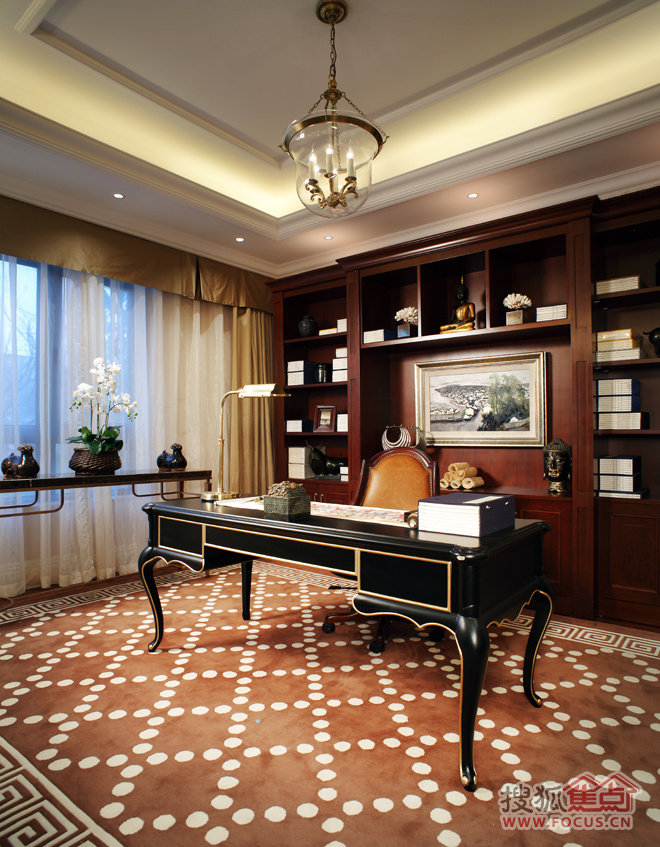设计TIPS：画品是中西贯通的表现手法，利用西方的工艺技巧表现了东方文化题材。奢华的单椅、边几、角几、壁纸与东方风情的画芯、茶杯图案、单椅软包巧妙的融合在一起，两种文化相互交织。

PART5：卧室设计设计TIPS：主卧室的面宽近15米，特别设计了一款中式屏风将主卧划为“休息区”与“书房区”，有效的利用了空间。坐在柔软的皮质沙发上，可以聊天、看电视。穿过屏风便是书房区域，在安静的卧室可以尽享恬静的阅读时光。。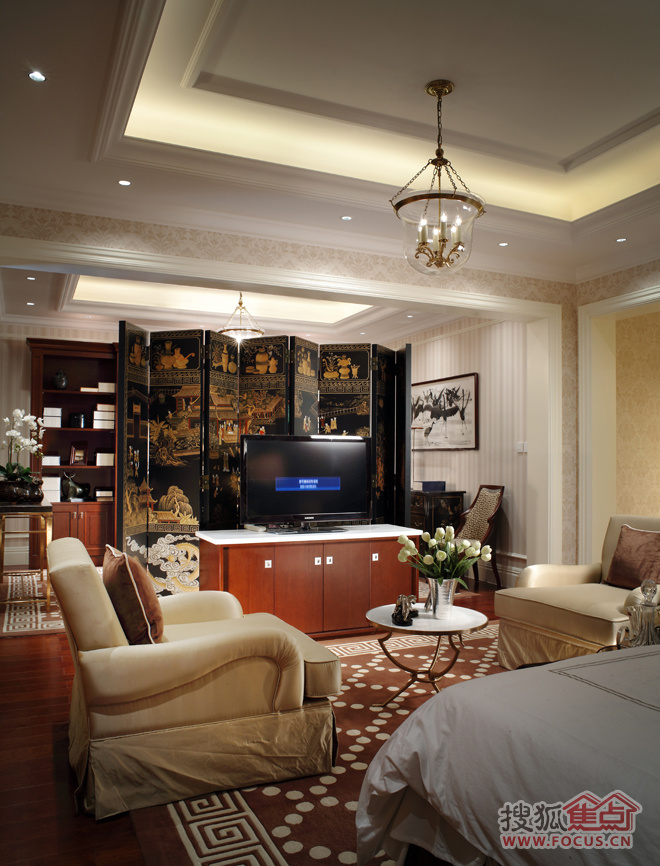设计TIPS：此处放置的充满东方韵味的“小叶紫檀屏风”与正对面的“法式文物柜”遥相呼应，展现了两种文化的交融。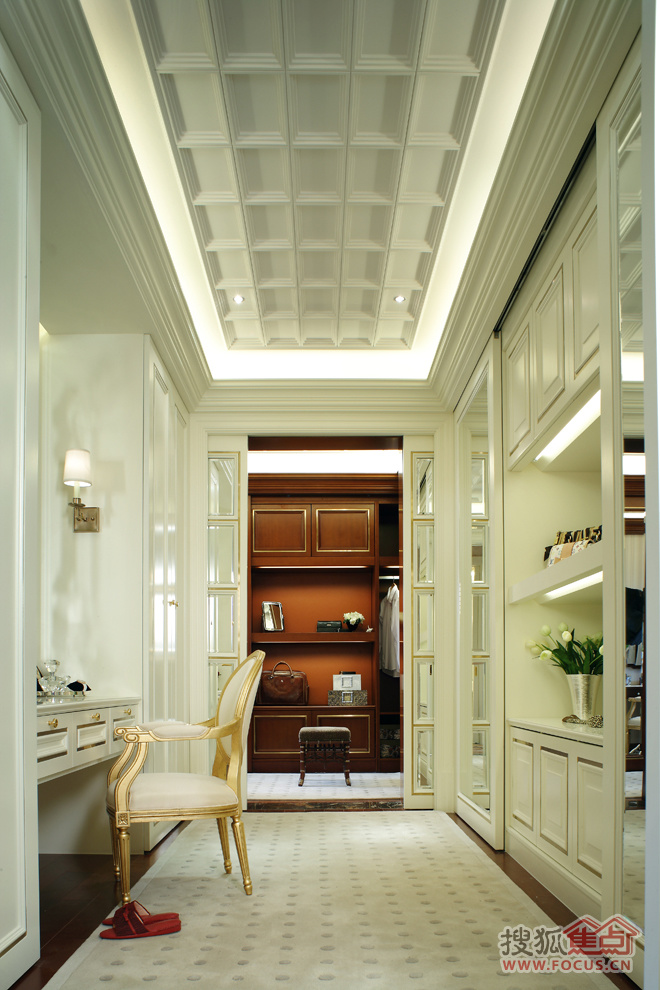设计TIPS：卧室入门处是一个“十字型”的分隔空间，右转进入“步入式衣帽间”。男女区域分开设计，女更衣室的设计灵感来自“CHANEL”展厅的更衣室设计，干净明亮的色彩、温暖的灯光、柔软的地毯，品味空间，感知到女性的精致、细腻格调。闭合推拉暗门后是一个整体，保持了女更衣间的完整性。吊顶与客厅一脉相承，使用“方格叠级”的吊顶，而顶面的方格与推拉门上的镜面造型处理同样展现了东西方文化的结合。打开推拉门后别有洞天：进入男士衣帽间，与女性衣帽间形成鲜明对比，在颜色上更体现男性的沉稳，质感，体现了设计的转折。。

PART6：儿童房设计设计TIPS：在主人房对面，是“儿童房”区域，为了丰富儿童的家庭生活，将空间设计为休息区、活动区。不论一位或者两位儿童，两个不同的功能空间都可以根据主人的需要，进行功能性的调整。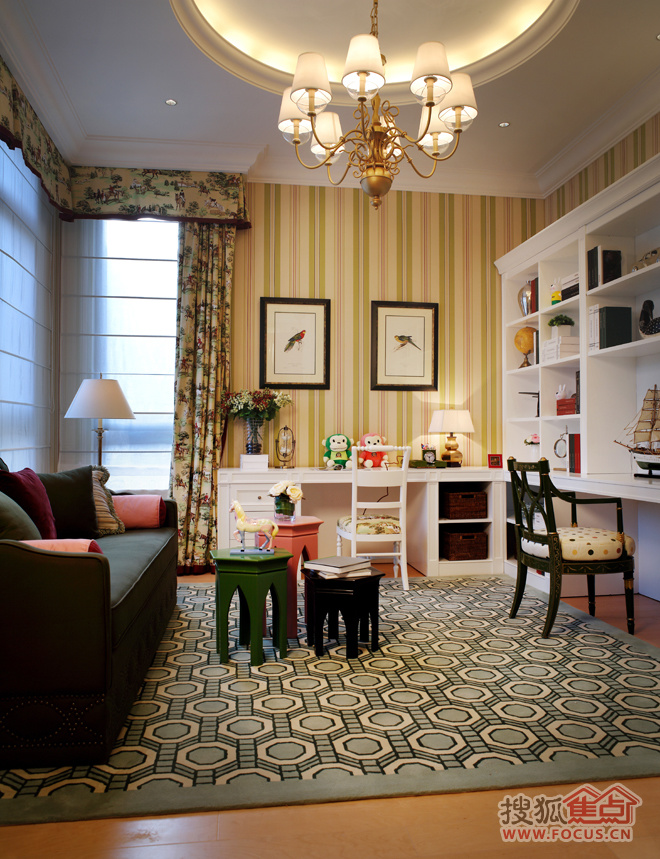设计TIPS：儿童房采用了强对比的红、绿色搭配，增加空间的活泼感，而休息区使用了东方窗棂图案演变的地毯图案、东方狩猎题材的窗帘图案等，不论从整体到细节，都可以欣赏到中西文化元素之间的巧妙交融。

PART7：浴室设计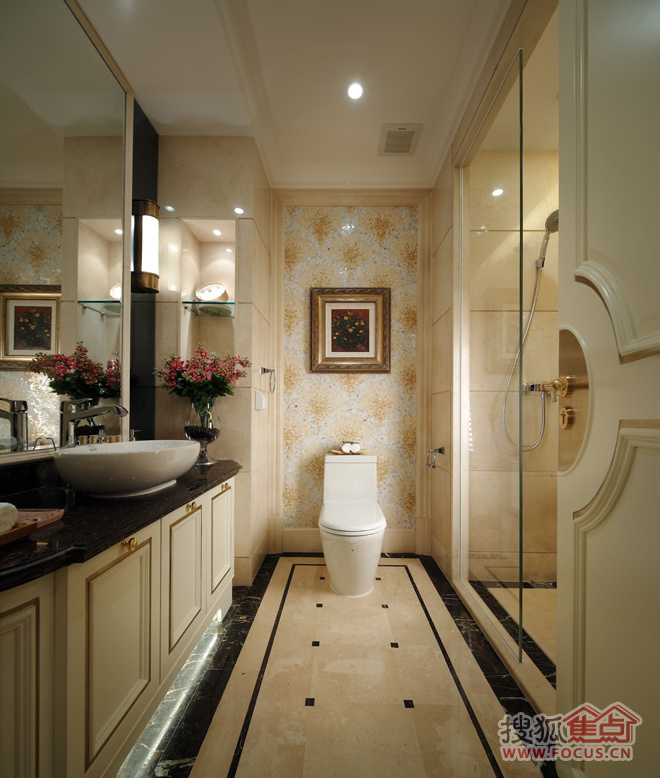设计TIPS：卧室“十字形”区域的左侧，是“主卧卫生间”。 卫生间的正面及两侧利用镜面明亮的“光折效果”做为墙面，对面同样也做了相同的设计，对称式的镜面设计在视觉上拉伸了整个空间的纵深感，空间变得更加明亮、开阔。

项目名称：伊泰华府世家

坐落地点：内蒙鄂尔多斯东胜区

设计公司：北京根尚国际空间设计有限公司

设计总监：王小根

设计师：彭迎芝、郭菲等

面积：560平米

51图晒1套房子的25种不同装修风格案例

76㎡温馨和风小户型 日本夫妇教你装出古朴范儿

8款乡村风格厨房案例 田园风厨房装修效果图

19图晒300平地中海+乡村混搭风格装修样板间

105平巧设计变3室2厅2卫1衣帽间北欧豪宅

`声明：本文由入驻焦点开放平台的作者撰写，除焦点官方账号外，观点仅代表作者本人，不代表焦点立场错误信息举报电话： 400-099-0099，邮箱：jubao@vip.sohu.com，或点此进行意见反馈，或点此进行举报投诉。`A B C D E F G H J K L M N P Q R S T W X Y Z
A - B - C - D - E
• A
• 鞍山
• 安庆
• 安阳
• 安顺
• 安康
• 澳门
• B
• 北京
• 保定
• 包头
• 巴彦淖尔
• 本溪
• 蚌埠
• 亳州
• 滨州
• 北海
• 百色
• 巴中
• 毕节
• 保山
• 宝鸡
• 白银
• 巴州
• C
• 承德
• 沧州
• 长治
• 赤峰
• 朝阳
• 长春
• 常州
• 滁州
• 池州
• 长沙
• 常德
• 郴州
• 潮州
• 崇左
• 重庆
• 成都
• 楚雄
• 昌都
• 慈溪
• 常熟
• D
• 大同
• 大连
• 丹东
• 大庆
• 东营
• 德州
• 东莞
• 德阳
• 达州
• 大理
• 德宏
• 定西
• 儋州
• 东平
• E
• 鄂尔多斯
• 鄂州
• 恩施
F - G - H - I - J
• F
• 抚顺
• 阜新
• 阜阳
• 福州
• 抚州
• 佛山
• 防城港
• G
• 赣州
• 广州
• 桂林
• 贵港
• 广元
• 广安
• 贵阳
• 固原
• H
• 邯郸
• 衡水
• 呼和浩特
• 呼伦贝尔
• 葫芦岛
• 哈尔滨
• 黑河
• 淮安
• 杭州
• 湖州
• 合肥
• 淮南
• 淮北
• 黄山
• 菏泽
• 鹤壁
• 黄石
• 黄冈
• 衡阳
• 怀化
• 惠州
• 河源
• 贺州
• 河池
• 海口
• 红河
• 汉中
• 海东
• 怀来
• I
• J
• 晋中
• 锦州
• 吉林
• 鸡西
• 佳木斯
• 嘉兴
• 金华
• 景德镇
• 九江
• 吉安
• 济南
• 济宁
• 焦作
• 荆门
• 荆州
• 江门
• 揭阳
• 金昌
• 酒泉
• 嘉峪关
K - L - M - N - P
• K
• 开封
• 昆明
• 昆山
• L
• 廊坊
• 临汾
• 辽阳
• 连云港
• 丽水
• 六安
• 龙岩
• 莱芜
• 临沂
• 聊城
• 洛阳
• 漯河
• 娄底
• 柳州
• 来宾
• 泸州
• 乐山
• 六盘水
• 丽江
• 临沧
• 拉萨
• 林芝
• 兰州
• 陇南
• M
• 牡丹江
• 马鞍山
• 茂名
• 梅州
• 绵阳
• 眉山
• N
• 南京
• 南通
• 宁波
• 南平
• 宁德
• 南昌
• 南阳
• 南宁
• 内江
• 南充
• P
• 盘锦
• 莆田
• 平顶山
• 濮阳
• 攀枝花
• 普洱
• 平凉
Q - R - S - T - W
• Q
• 秦皇岛
• 齐齐哈尔
• 衢州
• 泉州
• 青岛
• 清远
• 钦州
• 黔南
• 曲靖
• 庆阳
• R
• 日照
• 日喀则
• S
• 石家庄
• 沈阳
• 双鸭山
• 绥化
• 上海
• 苏州
• 宿迁
• 绍兴
• 宿州
• 三明
• 上饶
• 三门峡
• 商丘
• 十堰
• 随州
• 邵阳
• 韶关
• 深圳
• 汕头
• 汕尾
• 三亚
• 三沙
• 遂宁
• 山南
• 商洛
• 石嘴山
• T
• 天津
• 唐山
• 太原
• 通辽
• 铁岭
• 泰州
• 台州
• 铜陵
• 泰安
• 铜仁
• 铜川
• 天水
• 天门
• W
• 乌海
• 乌兰察布
• 无锡
• 温州
• 芜湖
• 潍坊
• 威海
• 武汉
• 梧州
• 渭南
• 武威
• 吴忠
• 乌鲁木齐
X - Y - Z
• X
• 邢台
• 徐州
• 宣城
• 厦门
• 新乡
• 许昌
• 信阳
• 襄阳
• 孝感
• 咸宁
• 湘潭
• 湘西
• 西双版纳
• 西安
• 咸阳
• 西宁
• 仙桃
• 西昌
• Y
• 运城
• 营口
• 盐城
• 扬州
• 鹰潭
• 宜春
• 烟台
• 宜昌
• 岳阳
• 益阳
• 永州
• 阳江
• 云浮
• 玉林
• 宜宾
• 雅安
• 玉溪
• 延安
• 榆林
• 银川
• Z
• 张家口
• 镇江
• 舟山
• 漳州
• 淄博
• 枣庄
• 郑州
• 周口
• 驻马店
• 株洲
• 张家界
• 珠海
• 湛江
• 肇庆
• 中山
• 自贡
• 资阳
• 遵义
• 昭通
• 张掖
• 中卫

1室1厅1厨1卫1阳台

1
2
3
4
5

0
1
2

1

1

0
1
2
3报名成功，资料已提交审核A B C D E F G H J K L M N P Q R S T W X Y Z
A - B - C - D - E
• A
• 鞍山
• 安庆
• 安阳
• 安顺
• 安康
• 澳门
• B
• 北京
• 保定
• 包头
• 巴彦淖尔
• 本溪
• 蚌埠
• 亳州
• 滨州
• 北海
• 百色
• 巴中
• 毕节
• 保山
• 宝鸡
• 白银
• 巴州
• C
• 承德
• 沧州
• 长治
• 赤峰
• 朝阳
• 长春
• 常州
• 滁州
• 池州
• 长沙
• 常德
• 郴州
• 潮州
• 崇左
• 重庆
• 成都
• 楚雄
• 昌都
• 慈溪
• 常熟
• D
• 大同
• 大连
• 丹东
• 大庆
• 东营
• 德州
• 东莞
• 德阳
• 达州
• 大理
• 德宏
• 定西
• 儋州
• 东平
• E
• 鄂尔多斯
• 鄂州
• 恩施
F - G - H - I - J
• F
• 抚顺
• 阜新
• 阜阳
• 福州
• 抚州
• 佛山
• 防城港
• G
• 赣州
• 广州
• 桂林
• 贵港
• 广元
• 广安
• 贵阳
• 固原
• H
• 邯郸
• 衡水
• 呼和浩特
• 呼伦贝尔
• 葫芦岛
• 哈尔滨
• 黑河
• 淮安
• 杭州
• 湖州
• 合肥
• 淮南
• 淮北
• 黄山
• 菏泽
• 鹤壁
• 黄石
• 黄冈
• 衡阳
• 怀化
• 惠州
• 河源
• 贺州
• 河池
• 海口
• 红河
• 汉中
• 海东
• 怀来
• I
• J
• 晋中
• 锦州
• 吉林
• 鸡西
• 佳木斯
• 嘉兴
• 金华
• 景德镇
• 九江
• 吉安
• 济南
• 济宁
• 焦作
• 荆门
• 荆州
• 江门
• 揭阳
• 金昌
• 酒泉
• 嘉峪关
K - L - M - N - P
• K
• 开封
• 昆明
• 昆山
• L
• 廊坊
• 临汾
• 辽阳
• 连云港
• 丽水
• 六安
• 龙岩
• 莱芜
• 临沂
• 聊城
• 洛阳
• 漯河
• 娄底
• 柳州
• 来宾
• 泸州
• 乐山
• 六盘水
• 丽江
• 临沧
• 拉萨
• 林芝
• 兰州
• 陇南
• M
• 牡丹江
• 马鞍山
• 茂名
• 梅州
• 绵阳
• 眉山
• N
• 南京
• 南通
• 宁波
• 南平
• 宁德
• 南昌
• 南阳
• 南宁
• 内江
• 南充
• P
• 盘锦
• 莆田
• 平顶山
• 濮阳
• 攀枝花
• 普洱
• 平凉
Q - R - S - T - W
• Q
• 秦皇岛
• 齐齐哈尔
• 衢州
• 泉州
• 青岛
• 清远
• 钦州
• 黔南
• 曲靖
• 庆阳
• R
• 日照
• 日喀则
• S
• 石家庄
• 沈阳
• 双鸭山
• 绥化
• 上海
• 苏州
• 宿迁
• 绍兴
• 宿州
• 三明
• 上饶
• 三门峡
• 商丘
• 十堰
• 随州
• 邵阳
• 韶关
• 深圳
• 汕头
• 汕尾
• 三亚
• 三沙
• 遂宁
• 山南
• 商洛
• 石嘴山
• T
• 天津
• 唐山
• 太原
• 通辽
• 铁岭
• 泰州
• 台州
• 铜陵
• 泰安
• 铜仁
• 铜川
• 天水
• 天门
• W
• 乌海
• 乌兰察布
• 无锡
• 温州
• 芜湖
• 潍坊
• 威海
• 武汉
• 梧州
• 渭南
• 武威
• 吴忠
• 乌鲁木齐
X - Y - Z
• X
• 邢台
• 徐州
• 宣城
• 厦门
• 新乡
• 许昌
• 信阳
• 襄阳
• 孝感
• 咸宁
• 湘潭
• 湘西
• 西双版纳
• 西安
• 咸阳
• 西宁
• 仙桃
• 西昌
• Y
• 运城
• 营口
• 盐城
• 扬州
• 鹰潭
• 宜春
• 烟台
• 宜昌
• 岳阳
• 益阳
• 永州
• 阳江
• 云浮
• 玉林
• 宜宾
• 雅安
• 玉溪
• 延安
• 榆林
• 银川
• Z
• 张家口
• 镇江
• 舟山
• 漳州
• 淄博
• 枣庄
• 郑州
• 周口
• 驻马店
• 株洲
• 张家界
• 珠海
• 湛江
• 肇庆
• 中山
• 自贡
• 资阳
• 遵义
• 昭通
• 张掖
• 中卫• 手机• 分享
• 设计
免费设计
• 计算器
装修计算器
• 入驻
合作入驻
• 联系
联系我们
• 置顶
返回顶部